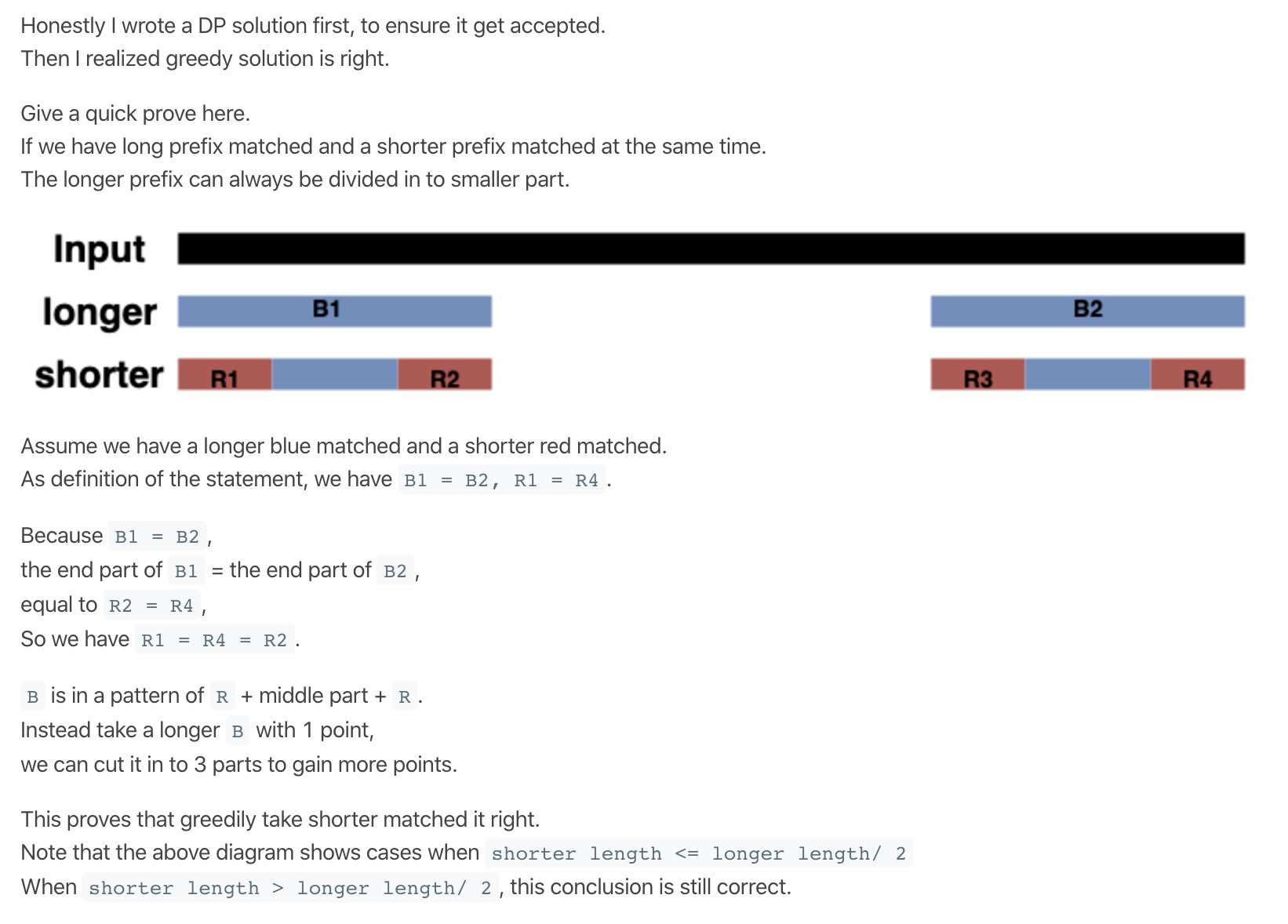# LeetCode1147-段式回文

### 段式回文

Difficulty: 困难

• 每个 `a_i` 都是一个非空字符串；
• 将这些字符串首位相连的结果 `a_1 + a_2 + ... + a_k` 和原始字符串 `text` 相同；
• 对于所有`1 <= i <= k`，都有 `a_i = a_{k+1 - i}`

``````输入：text = "ghiabcdefhelloadamhelloabcdefghi"

``````

``````输入：text = "merchant"

``````

``````输入：text = "antaprezatepzapreanta"

``````

``````输入：text = "aaa"

``````

• `text` 仅由小写英文字符组成。
• `1 <= text.length <= 1000`

#### Solution

Language: C++

``````class Solution {
public:
int longestDecomposition(string text) {
int n = text.size();
if(n == 0)
return 0;
for(int i = 1;i <= n/2; ++i){
if(text.substr(0,i) == text.substr(n-i,i)){
// 这里表示, 只要有一个最短匹配的就是最长的段式回文
return 2 + longestDecomposition(text.substr(i,n-2*i));
}
}
return 1;
}
};

``````

``````class Solution {
public:
int longestDecomposition(string text) {
int res = 0;
int prev = 0;
int S = text.size();
for (int i = 0; i < S / 2; ++i) {
if ((text.substr(prev, i - prev + 1)) == text.substr(S - 1 - i, i - prev + 1)) {
res += 2;
prev = i + 1;
}
}
// 中间剩余一个字母的情况: 奇数 或者 prev < S / 2 ,要累加1
if (S % 2 == 1 || prev < S / 2)
++res;
return res;
}
};

``````

``````int longestDecomposition(string S) {
int res = 0, n = S.length();
string l = "", r = "";
for (int i = 0; i < n; ++i) {
// 类似上面的截取字符串
l = l + S[i], r = S[n - i - 1] + r;
if (l == r)
++res, l = "", r = "";
}
return res;
}

``````

## 贪心证明